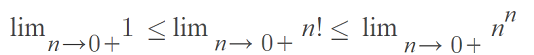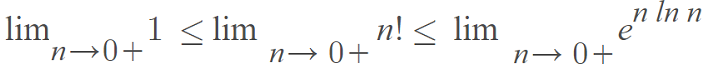# Zero Factorial: Why Does it Equal One?

If you’re wondering why zero factorial equals 1, you’re not alone. It’s one of the great puzzles of mathematics that seems completely unintuitive. There are a few different ways to show that 0! = 1 must be true:

## The Definition

The reason why 0! = 1 is because it’s defined that way.

“…zero factorial equals 1, 0! = 1 [and] 1! = 1. However, one cannot similarly conclude that 0 = 1 because the one result is arrived at by convention and the other result is a mathematical fact. As with all conventions certain protocols and rules must be followed in arithmetic operations involving zero” 

If you’re having trouble wrapping your head around that statement, you’re not alone. For millennia, mathematicians debated about whether or not zero (even without the factorial) existed at all!  The Roman Numeral system didn’t have zeros, and it wasn’t until the introduction of the decimal system in the 13th century that “zero” really became a thing. Still, working with “0” results in a lot of problems, including the unintuitive idea that 0! = 1.

## Showing 0! = 1 with the Combinations Formula

Note: You may want to read “What is a Factorial?” first.

We want to show that there’s only one way of choosing zero items (that zero factorial equals one) with the combinations formula from statistics:

• nCr = n! / ((n – r)! r!)

Where:

• n = Number of items.
• r = How many items are taken at a time.

Let’s start with three items and move backwards, so see if there’s a logical pattern.

Let’s say you had three novels on a shelf and you wanted to choose three at the same time. How many different ways could you grab all three at once? Logically, you’d say there’s only one way of grabbing all three. But you can show this with the combinations formula. We have n = 3 and r = 3, so:

3C3 = 3! / ((3 – 3)! 3!) = 1.

This is the answer we would expect. Now let’s try two books:

3C3 = 2! / (2 – 2)! 2! = 1.

Are you seeing the pattern? As long as you’re taking all of the items at the same time (we don’t care what order they are in), it’s always going to equal 1. You could put in 99,999 or 1 million and you would still get: 1.

Now we’ve established the logic, take a close look at one example:

• = 2! / (2 – 2)! 2!
• = 2 / (0)!*2

We know that this equation must equal two. The only way to make that happen is if 0! = 1. Otherwise you would have division by zero, which is not defined.

What happens if we follow our initial intuition and decide that 0! = 0? That would that the number of ways of choosing 3 books from a set of three would be undefined:

• = 3! / (3 – 3)! 3!
• = 6 / (0)*3 = undefined.

## Show that Zero Factorial Equals One With Limits

The following explanation  is fairly easy to follow with some basic calculus concepts.

1. Let’s define a factorial for items equal to or greater than one (we’re going to ignore 0! for a moment).
Definition: The factorial n is defined by n! = (n)(n – 1)…(3) (2) (1) for all n ≥ 1.
2. The lower bound for  must be 1, because 1n = (1) (1) (1) … → 1 ≤ n.
3. We can define an upper bound in the same way: n! ≤ (n) (n) (n) … = nn.
4. Putting (2) and (3) together, we get: 1 ≤ n! ≤ nn.
5. The upper bound in (4) is valid for n > 0, so take the limit as n → 0+:This limit is indeterminate because 0n = 0 for any n > 0 and n0 = 1 for any n ≠ 0.
6. From (5), we can say that7. Using L’Hospital’s rule, we have the limit of n ln n (as n→ 0+)
8. If we let the limit n! in (7) equal zero, we get: 1 ≤ 0! ≤ 1, which implies that 0! = 1.

## References

 Zero in Four Dimensions: Cultural, Historical, Mathematical, and Psychological Perspectives.
 Seife, C. (2000). Zero: The Biography of a Dangerous Idea. Penguin Books.
 Mahmood, M. & Mahmood, I. (2015). A simple demonstration of zero factorial equals one. International Journal of Mathematical Education 47(6):1-2

CITE THIS AS:
Stephanie Glen. "Zero Factorial: Why Does it Equal One?" From StatisticsHowTo.com: Elementary Statistics for the rest of us! https://www.statisticshowto.com/zero-factorial-why-does-it-equal-one/